• +91 9971497814
• info@interviewmaterial.com

# RD Chapter 23- The Straight Lines Ex-23.17 Interview Questions Answers

### Related Subjects

Question 1 :

Prove that the area of the parallelogram formed by the lines
a1x + b1y + c1 = 0, a1x + b1y+ d1 = 0, a2x + b2y + c2 =0, a2x + b2y + d2 = 0 is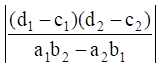sq. units.

Deduce the condition for these lines to form a rhombus.

Given:

The given lines are

a1x + b1y+ c1 = 0 … (1)

a1x + b1y+ d1 = 0 … (2)

a2x + b2y+ c2 = 0 … (3)

a2x + b2y+ d2 = 0 … (4)

Let us prove, the areaof the parallelogram formed by the lines a1x + b1y + c1 =0, a1x + b1y + d1 = 0, a2x + b2y+ c2 = 0, a2x + b2y + d2 =0 is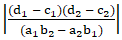sq. units.

The area of theparallelogram formed by the lines a1x + b1y + c1 =0, a1x + b1y + d1 = 0, a2x + b2y+ c2 = 0 and a2x + b2y + d2 =0 is given below: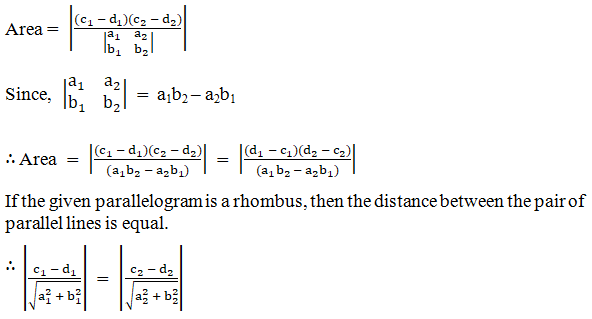Hence proved.

Question 2 : Prove that the area of the parallelogramformed by the lines 3x – 4y + a = 0, 3x –4y + 3a = 0, 4x – 3y – a = 0 and 4x –3y – 2a = 0 is 2a2/7 sq. units.

Given:

The given lines are

3x − 4y + a= 0 … (1)

3x − 4y + 3a= 0 … (2)

4x − 3y − a= 0 … (3)

4x − 3y − 2a= 0 … (4)

Let us prove, the areaof the parallelogram formed by the lines 3x – 4y + a = 0, 3x – 4y + 3a = 0, 4x– 3y – a = 0 and 4x – 3y – 2a = 0 is 2a2/7 sq. units.

From above solution,we know that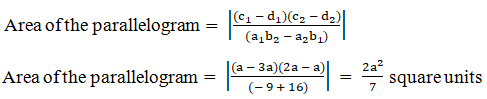Hence proved.

Question 3 :

Show that the diagonals of the parallelogram whose sides are lx + my + n= 0, lx + my + n’ = 0, mx + ly + n = 0 and mx + ly + n’ = 0 include an angleπ/2.

Given:

The given lines are

lx + my + n = 0 … (1)

mx + ly + n’ = 0 … (2)

lx + my + n’ = 0 … (3)

mx + ly + n = 0 … (4)

Let us prove, the diagonalsof the parallelogram whose sides are lx + my + n = 0, lx + my + n’ = 0, mx + ly+ n = 0 and mx + ly + n’ = 0 include an angle π/2.

By solving (1) and(2), we get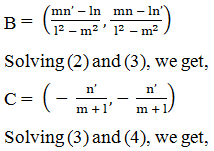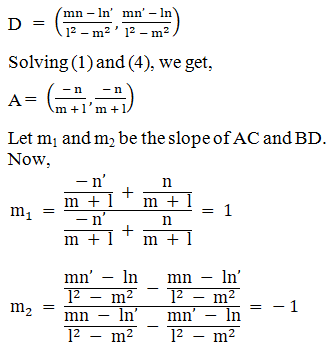m1m2 =-1

Hence proved.

Todays Deals### RD Chapter 23- The Straight Lines Ex-23.17 Contributorskrishan

Name:
Email:

# Latest News# 9000 interview questions in different categories